# Test: Displacement & Velocity

## 20 Questions MCQ Test Physics For JEE | Test: Displacement & Velocity

Description
Attempt Test: Displacement & Velocity | 20 questions in 20 minutes | Mock test for NEET preparation | Free important questions MCQ to study Physics For JEE for NEET Exam | Download free PDF with solutions
QUESTION: 1

### The displacement of a particle is given by x = (t – 2)2 where x is in meters and t in seconds. The distance covered by the particle in the first 4 seconds is:

Solution:

Displacement at t = 0 sec is: x = 4 meters
Displacement at t = 2 sec is: x = 0 meters
Displacement at t = 4 sec is: x = 22 = 4 meters
∵ Particle changes direction at t = 2s

⇒ Particle travels from x = 4 m to x = 0 m  in the first 2 sec and then  from x = 0 m to x = 4 m position in the next two seconds

Distance travelled = 8 meters.

QUESTION: 2

### The ratio of displacement to distance is:

Solution:
• Displacement is the shortest length between the starting and the end pointing of a journey, whereas the distance is the actual length of the path travelled.
• Hence, the distance will always be equal to or greater than the displacement.
• So, the ratio of displacement to distance will be always less than or equal to 1, since the denominator(distance) is either greater than or equal to the numerator (displacement).
QUESTION: 3

### A vehicle travels half the distance L with speed V1and the other half with speed V2, then its average speed is

Solution: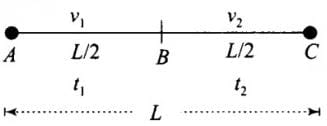Let the vehicle travels from A to B. Distances, velocities, and time taken are shown. To calculate average speed we will calculate the total distance covered and will divide it by the time interval in which it covers that total distance.

Time taken to travel first half distance t1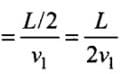Time taken to travel first half distance t1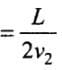Total time taken = t+ t2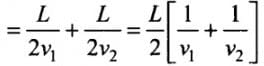We know that vav ​= Average speed = Total Distance / Total Time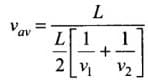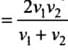QUESTION: 4

Which of the following statements about distance is true?

Solution:

Displacement is the net distance between the initial position and final position, while the distance is the measure of the total path traveled during the entire journey.

Thus, distance is always equal to or more than displacement.

QUESTION: 5

Which of the following quantity remains constant during the uniform circular motion?

Solution:

When a body is in a uniform circular motion, its speed remains constant but its velocity, angular acceleration, angular velocity change due to a change in its direction.

QUESTION: 6

The magnitude of average velocity is equal to the average speed if the motion of an object is along a straight line and in the same direction.

Solution:

The magnitude of the average velocity of an object is equal to its average speed, only in one condition when an object is moving in a straight line.

Therefore, the given statement is true.

QUESTION: 7

A particle is moving along a circle of radius R such that it completes one revolution in 40 seconds. What will be the displacement after 2 minutes 20 seconds?

Solution:

1 revolution in 40 seconds
In 1 second it covers = 1/40 revolution
In 140 sec = 1/40 * 140 =7/2 rotation = 3 and half rotation

Then the particle will be on the diametrically opposite end.

Therefore, Displacement = R + R = 2R

QUESTION: 8

The numerical ratio of velocity to speed is:

Solution:
• When an object is moving along a straight path, the magnitude of average velocity is equal to the average speed.
• Therefore, the numerical ratio of average velocity to average speed is one in a straight line motion.
• But, during curved motion, the displacement < distance covered, so the velocity < speed.

Therefore, the numerical ratio of average velocity to average speed is equal to or less than one but can never be more than one.

QUESTION: 9

Two escalators move people up and down between floors of a shopping mall at the same rate, either up or down. What of the following statements regarding the motion of the escalators is true?

Solution:

As the particles are moving at the same rate, their speed is the same but velocities will be different beacuse their directions will be different.

QUESTION: 10

A coordinate system that enables us to describe the position of a body in space at any instant of time is called:

Solution:

A coordinate system that enables us to describe the position of a body in space at any instant of time is called the Origin Reference point or Intersection point or Reference frame

QUESTION: 11

The displacement of a particle starting from rest (at t = 0) is given by s = 6t2-t. The time in seconds at which the particle will attain zero velocity again, is

Solution:

v = ds/dt

v = 12t - 3t2

0 = 3t (4 - t)
⇒ t = 0 or 4
Here t = 0 is not realistic so at t = 4 the velocity will be zero.

QUESTION: 12

The displacement of an object may be:

Solution:

The displacement is a relative term, therefore it can be positive, negative or even 0 depending upon the initial position.

QUESTION: 13

If a body does not change its direction during the course of its motion, then ______.

Solution:

Since the object does not change its direction it means that the object is traveling in a straight line.
⇒ The path length and displacement will be equal.

QUESTION: 14

36 km/h is equal to:

Solution: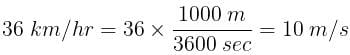QUESTION: 15

The ratio of magnitude of velocity and speed is always:

Solution:

The displacement of the body at any given time is always equal to or less than the distance covered.
∵ Velocity is displacement per unit time and speed is the distance covered per unit time.
∴ The ratio of the magnitude of velocity and speed is always equal to or less than one.

QUESTION: 16

Suppose our school is 1 km away from our house, and we go to school in the morning, and in the afternoon we come back. Then, the total displacement for the entire trip is:

Solution:
• Displacement quantifies both direction and distance of an imaginary motion along a straight line from the initial position to the final position of the point.
• Here, since the same amount of distance is covered in the opposite direction, the total displacement for the entire trip is zero.
QUESTION: 17

An object is in uniform motion along a straight line if it:

Solution:
• Uniform motion means regular or equal motion.
• Thus uniform motion is obtained when an object travels an equal distance in equal intervals of time.
• Examples of uniform motion are a car moving with constant velocity, a boy running in a straight path with constant speed etc.
QUESTION: 18

A man starts from his home with a speed of 4 km/h on a straight road up to his office 5 km away and returns to home, then the path length covered is:

Solution:

5 km while going and 5 km while coming back.
⇒ Total path travelled = 10 km

QUESTION: 19

A car covers a distance of 5 km in 5 mins, its average speed is equal to:

Solution:

5 km in 5 mins, which means 1 km in 1 min
Hence, it will travel 60 km in 60 mins, i.e. speed = 60km/hr

QUESTION: 20

Which of the following is not an example of a rectilinear motion?

Solution:

Rectilinear motion is another name for straight-line motion
A body is said to experience rectilinear motion if any two particles of the body travel the same distance along two parallel straight lines.

A car moving in a circular path changes its direction at every instant. Thus, it is not the rectilinear motion.Use Code STAYHOME200 and get INR 200 additional OFF Use Coupon Code